Nonhyperbolic reflection moveout of-waves: An overview and comparison of reasonsNext: HORIZONTAL REFLECTOR BENEATH A Up: Fomel & Grechka: Nonhyperbolic Previous: Introduction

# WEAK ANISOTROPY APPROXIMATION for VTI media

In transversely isotropic media, velocities of seismic waves depend on the direction of propagation measured from the symmetry axis. Thomsen (1986) introduced a notation for VTI media by replacing the elastic stiffness coefficients with the- and-wave velocities along the symmetry axis and three dimensionless anisotropic parameters. As shown by Tsvankin (Tsvankin, 1996), the-wave seismic signatures in VTI media can be conveniently expressed in terms of Thomsen's parametersand. Deviations of these parameters from zero characterize the relative strength of anisotropy. For small values of these parameters,the weak-anisotropy approximation (Thomsen, 1986; Tsvankin and Thomsen, 1994) reduces to simple linearization.

The squared group velocityof-waves in weakly anisotropic VTI media can be expressed as a function of the group anglemeasured from the vertical symmetry axis as follows:(1)

where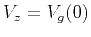is the-wave vertical velocity, andandare Thomsen's dimensionless anisotropic parameters, which are assumed to be small quantities:(2)

Both parameters are equal to zero in isotropic media.

Equation (1) is accurate up to the second-order terms inand. We retain this level of accuracy throughout the paper. As follows from equation (1), the velocitycorresponding to ray propagation in the horizontal direction is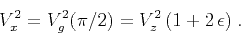(3)

Equation (3) is actually exact, valid for any strength of anisotropy. Another important quantity is the normal-moveout (NMO) velocity,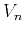, that determines the small-offset-wave reflection moveout in homogeneous VTI media above a horizontal reflector. Its exact expression is (Thomsen, 1986)(4)

Ifas, for example, in the ANNIE model proposed by Schoenberg et al. (1996), the normal-moveout velocity is equal to the vertical velocity.

It is convenient to rewrite equation (1) in the form(5)

where(6)

Equation (6) is the weak-anisotropy approximation for the anellipticitycoefficientintroduced by Alkhalifah and Tsvankin (1995). For the elliptic anisotropy,and. To see why the group-velocity function becomes elliptic in this case, note that for small(7)

Seismic data often indicate that, so the anellipticity coefficientis usually positive.

An equivalent form of equation (1) can be obtained in terms of the three characteristic velocities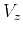,, and:(8)

From equation (8), in the linear approximation the anelliptic behavior of velocity is controlled by the difference between the normal moveout and horizontal velocities or, equivalently, by the difference between anisotropic coefficientsand.

We illustrate different types of the group velocities (wavefronts) in Figure 1. The wavefront, circular in the isotropic case (Figure 1a), becomes elliptical when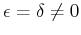(Figure 1b). In the ANNIE model, the vertical and NMO velocities are equal (Figure 1c). Ifand, the three characteristic velocities satisfy the inequality(Figure 1d).nmofro
Figure 1.
Wavefronts in isotropic medium,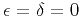(a), elliptically anisotropic medium,(b), ANNIE model,,(c), and anisotropic medium with,(d). Solid curves represent the wavefronts. Dashed lines correspond to isotropic wavefronts for the vertical and horizontal velocities.Nonhyperbolic reflection moveout of-waves: An overview and comparison of reasonsNext: HORIZONTAL REFLECTOR BENEATH A Up: Fomel & Grechka: Nonhyperbolic Previous: Introduction

2014-01-27